Home

# Impact of the variations of the mixing length in a first order turbulent closure system

## Abstract

This paper is devoted to the study of a turbulent circulation model. Equations are derived from the “Navier-Stokes turbulent kinetic energy” system. Some simplifications are performed but attention is focused on non linearities linked to turbulent eddy viscosity  $\nu _{t}$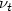. The mixing length $\ell$acts as a parameter which controls the turbulent part in $\nu _{t}$. The main theoretical results that we have obtained concern the uniqueness of the solution for bounded eddy viscosities and small values of $\ell$and its asymptotic decreasing as $\ell \rightarrow \infty$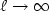in more general cases. Numerical experiments illustrate but also allow to extend these theoretical results: uniqueness is proved only for $\ell$small enough while regular solutions are numerically obtained for any values of $\ell$. A convergence theorem is proved for turbulent kinetic energy: $k_{\ell }\rightarrow 0$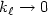as $\ell \rightarrow \infty ,$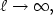but for velocity $u_{\ell }$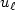we obtain only weaker results. Numerical results allow to conjecture that $k_{\ell }\rightarrow 0,$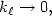$\nu _{t}\rightarrow \infty$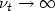and $u_{\ell }\rightarrow 0$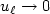as $\ell \rightarrow \infty .$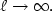So we can conjecture that this classical turbulent model obtained with one degree of closure regularizes the solution.

Hide All

# Impact of the variations of the mixing length in a first order turbulent closure system

## Metrics

### Full text viewsFull text views reflects the number of PDF downloads, PDFs sent to Google Drive, Dropbox and Kindle and HTML full text views.

Total number of HTML views: 0
Total number of PDF views: 0 *Loading metrics...

### Abstract viewsAbstract views reflect the number of visits to the article landing page.

Total abstract views: 0 *Loading metrics...

* Views captured on Cambridge Core between <date>. This data will be updated every 24 hours.

Usage data cannot currently be displayed.